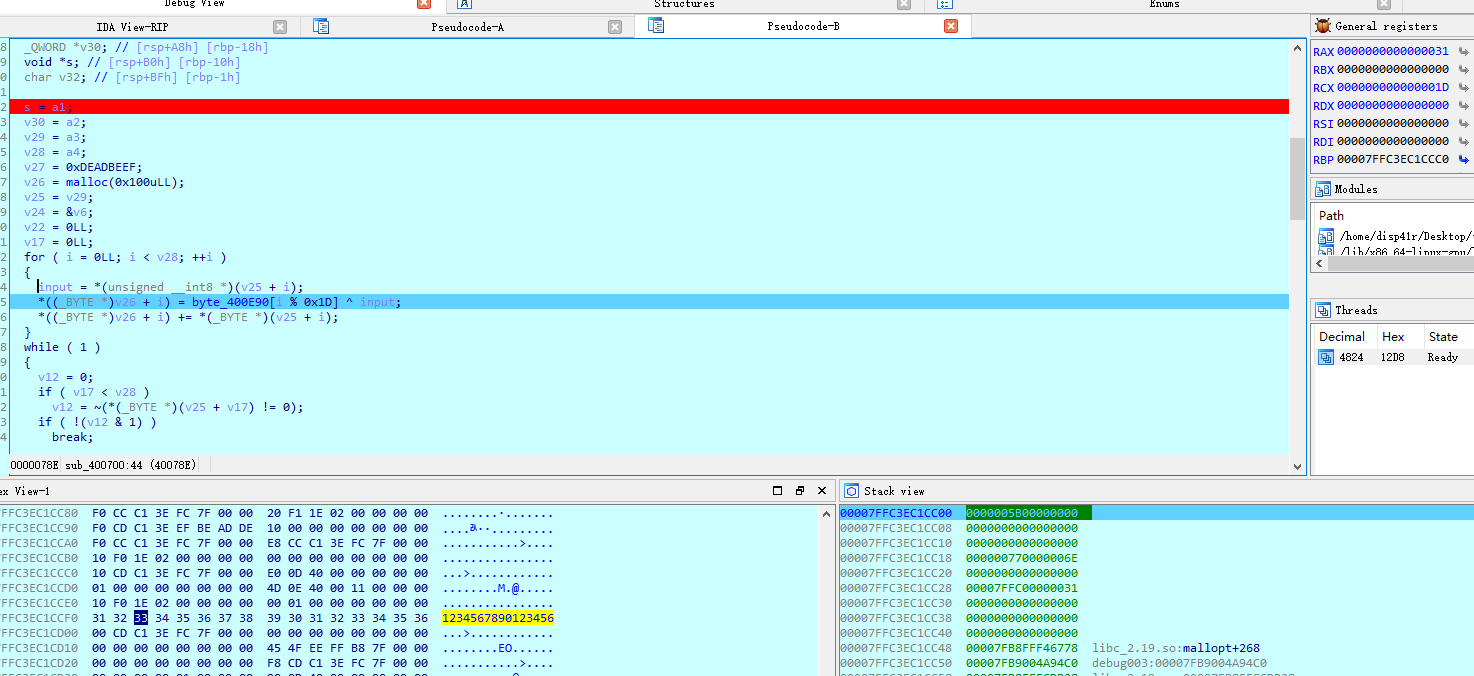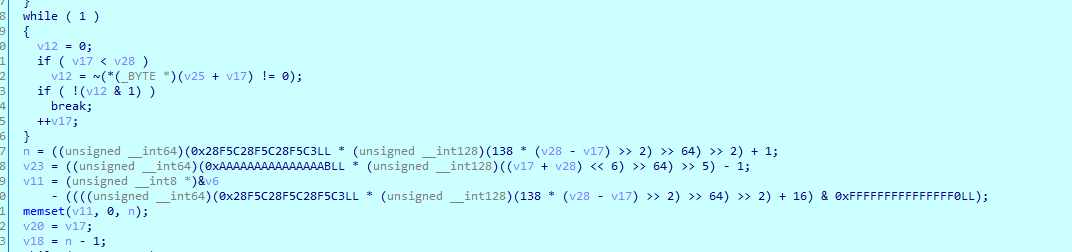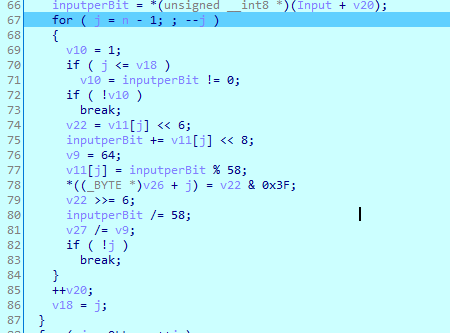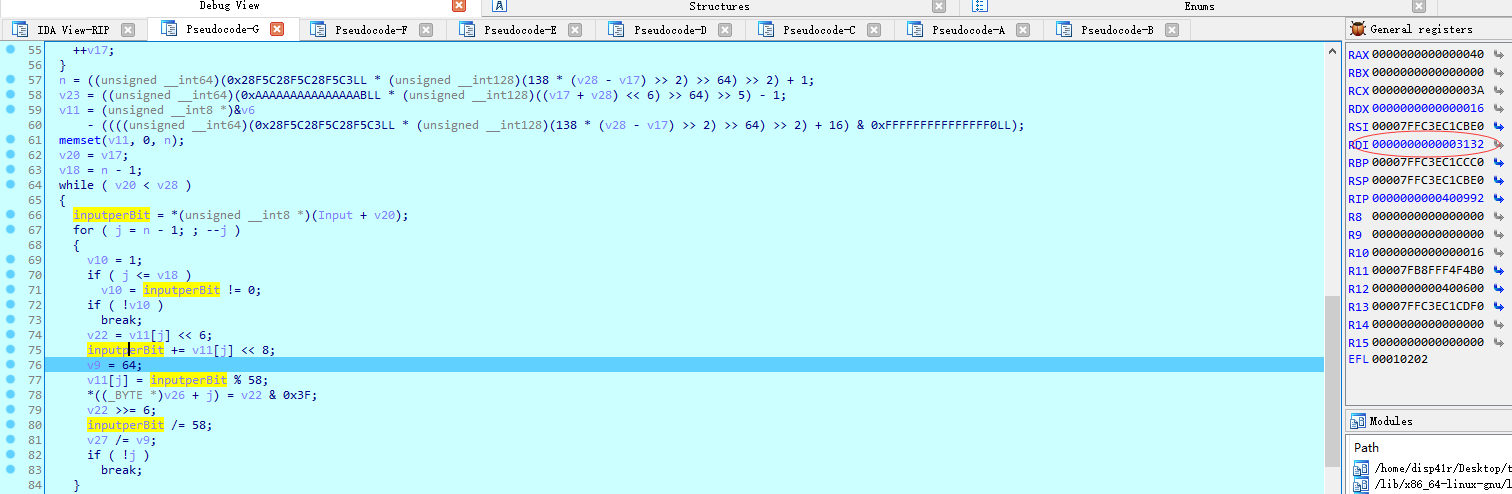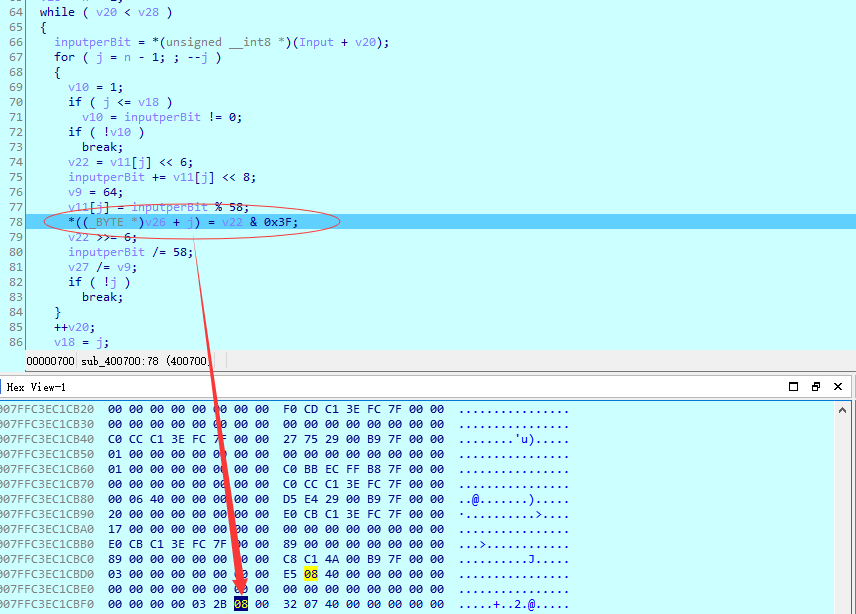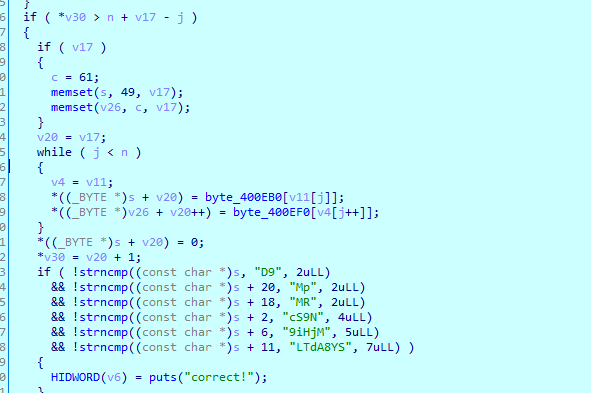## confusing base58

https://www.jianshu.com/p/5c59c45d144e 这篇文章对base58做了比较详细的解释，大概就是，出入的string-》转byte流-》转大整数-》转58进制数（不断对58取余）-》去base58Table里对应的字符。

``````__b58chars = '123456789ABCDEFGHJKLMNPQRSTUVWXYZabcdefghijkmnopqrstuvwxyz'
__b58base = len(__b58chars)
def b58encode(v):
long_value = int(v.encode("hex_codec"), 16)#输入转大整数
result = ''
while long_value >= __b58base:
div, mod = divmod(long_value, __b58base)#不断对58取余，也就是转58进制的过程
result = __b58chars[mod] + result
long_value = div
result = __b58chars[long_value] + result
# Bitcoin does a little leading-zero-compression:
# leading 0-bytes in the input become leading-1s
nPad = 0
for c in v:
if c == '\0':#填充过程
nPad += 1
else:
break
return (__b58chars * nPad) + result
def b58decode(v):
""" decode v into a string of len bytes
"""
long_value = 0L
for (i, c) in enumerate(v[::-1]):
long_value += __b58chars.find(c) * (__b58base ** i)#根据在表中的下标生成原本的58进制数
result = ''
while long_value >= 256:
div, mod = divmod(long_value, 256)#58进制转hex过程
result = chr(mod) + result
long_value = div
result = chr(long_value) + result
nPad = 0
for c in v:
if c == __b58chars:
nPad += 1
else:
break
result = chr(0) * nPad + result
return result
``````